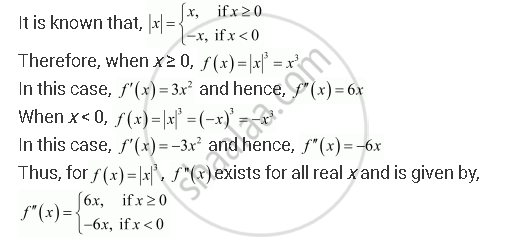Share

# If F (X) = |X|3, Show that F ″(X) Exists for All Real X and Find It. - CBSE (Science) Class 12 - Mathematics

#### Question

If f (x) = |x|3, show that f ″(x) exists for all real x and find it.

#### SolutionIs there an error in this question or solution?

#### APPEARS IN

NCERT Solution for Mathematics Textbook for Class 12 (2018 to Current)
Chapter 5: Continuity and Differentiability
Q: 18 | Page no. 192

#### Video TutorialsVIEW ALL 

Solution If F (X) = |X|3, Show that F ″(X) Exists for All Real X and Find It. Concept: Concept of Differentiability.
S